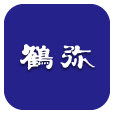# Tsuruya Co Ltd (TSE:5386)

398 JPY -3 JPY ( -0.75% )Tsuruya Co Ltd
TSE:5386

-

-

## FAQ

### What is Discount Rate?

Discount rate reflects the opportunity cost of investment (i.e. the return that could be earned on investment with similar risk).

### Cost of Equity Calculation

Cost of Equity
-
Rf
-
Beta
-
ERP
-

The Cost of Equity for Tsuruya Co Ltd (TSE:5386) calculated via CAPM (Capital Asset Pricing Model) is -.

### WACC Calculation

WACC
-

Cost of Equity
-
Equity Weight
-

Cost of Debt
-
Debt Weight
-

The WACC for Tsuruya Co Ltd (TSE:5386) is -.